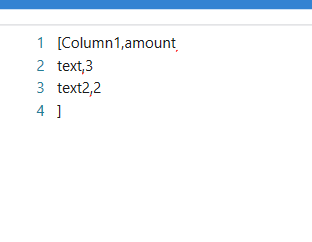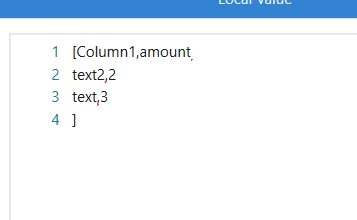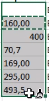# Select to sort in ascending order a datatable

Hi all,

I am trying to ascending sort a column (general type in excel) from a datatable, with the following expression:

(From x In dt.AsEnumerable() Order By convert.ToDouble(x(“amount”)) Select x).CopyToDataTable

Input string is not in the correct form. Any help?

@Kumar802

`dt.AsEnumerable.OrderBy(function(x) If(IsNumeric(x("amount")),CDBL(x("Amount").ToString.Trim),0)).CopyToDataTable`

If the given string is blank or not in numeric format we might get that error…so first I am checking if it is numeric else assigning 0

cheers

1 Like

Shown this error:

cannot be inferred from these arguments. Specifying the data type explictly might correct this error

@Kumar802

Can you show what you tried…As I did the same and can get the output as expected

Initial dataUsed assign with above formula

OutputOr Alternately try moving IsNumeric to last

`dt.AsEnumerable.OrderBy(function(x) If(x("amount").ToString.Trim.IsNumeric,CDBL(x("Amount").ToString.Trim),0)).CopyToDataTable`

cheers

1 Like

Hi @Kumar802 ,

Could you check with the below Expression :

``````DT.AsEnumerable.OrderBy(Function(x) If(x("Amount").ToString.IsNumeric,CDbl(x("Amount").ToString.Trim),0)).CopyToDataTable
``````

This is the input excel fileThis is the output with the expression:

blank
400
“70,7”
“493,5”
“160,00”
“169,00”
“295,00”

I can’t figure out what’s wrong.

@Kumar802

I guess it is because of decimal places being separated with comma

`DT.AsEnumerable.OrderBy(Function(x) If(x("Amount").ToString.Replace(",",".").IsNumeric,CDbl(x("Amount").ToString.Trim.Replace(",",".")),0)).CopyToDataTable`

cheers

@Kumar802

As the culture format of number is different instead of replace you can as well use tryparse to parse with culture of comma as decimals

`dt.AsEnumerable.OrderBy(function(x) If(Double.TryParse(x("amount").ToString,NumberStyles.Number,CultureInfo.GetCultureInfo("it-IT"),0),Double.Parse(x("amount").ToString,NumberStyles.Number,CultureInfo.GetCultureInfo("it-IT")),0)).CopyToDataTable`

Please let me know if you face issues

cheers